# Blog

## icon

Reference## 套版

Pinterest.com## [R] 字串操作：Regular Expression 及 stringr 套件

There are some handy functions in the package `stringr` to be substituted for lousy base R string manipulation functions.

### Functions for string manipulation in base funciton and `stringr` package

stringr base Description
`str_match` `regmaches` + `regexpr` Extract matched groups from a string
`str_match_all` `regmaches` + `gregexpr` Extract matched groups from a string (globally)
`str_replace` `sub` Replace first matched patterns in a string
`str_replace_all` `gsub` Replace all matched patterns in a string
`str_detect` `grepl` Detect the presence or absence of a pattern in a string
`str_subset` `grep(value = TRUE)` x[str_detect(x, pattern)]
`str_split` `strsplit` Split up a string into pieces
`str_length` `nchar` The length of a string
`str_sub` `substr` Extract and replace substrings from a character vector

## 在 RStudio 檢視 xml/html 的工具：xmlview Package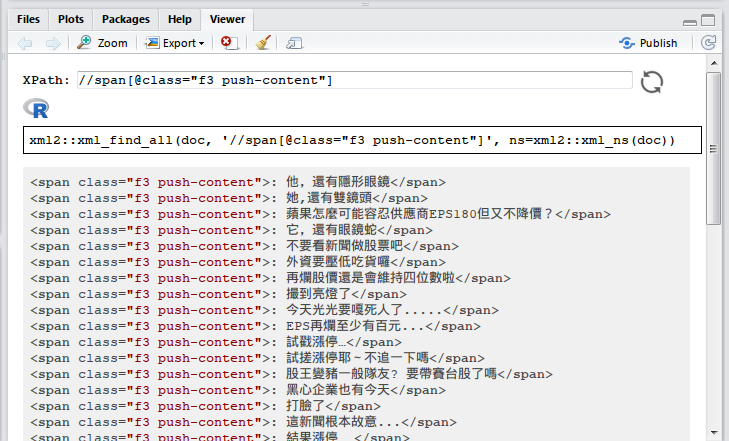`xmlview` package 提供了一個在 RStudio 上互動檢視 XML 以及測試 XPath 的方式，這裡用個簡單的 XML 當例子：

``````# devtools::install_github("hrbrmstr/xmlview")
library(xml2)
library(xmlview)
library(magrittr)

## plain text XML
``````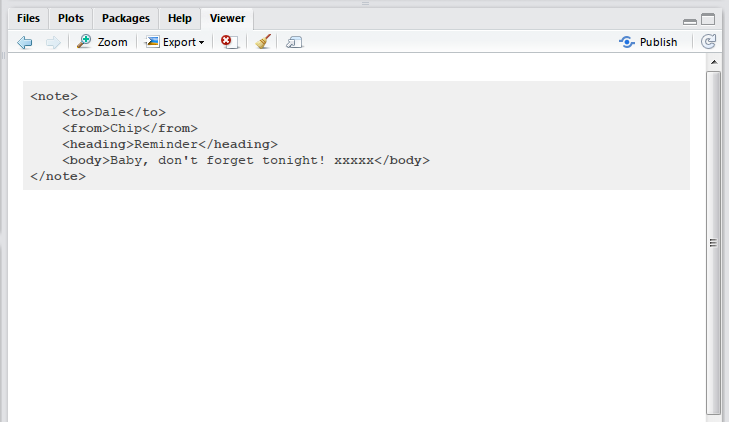### XPath 測試

``````## read-in XML document
encoding = "UTF-8")
``````

``````doc_string <- as.character(doc) %>% `Encoding<-`("UTF-8")
``````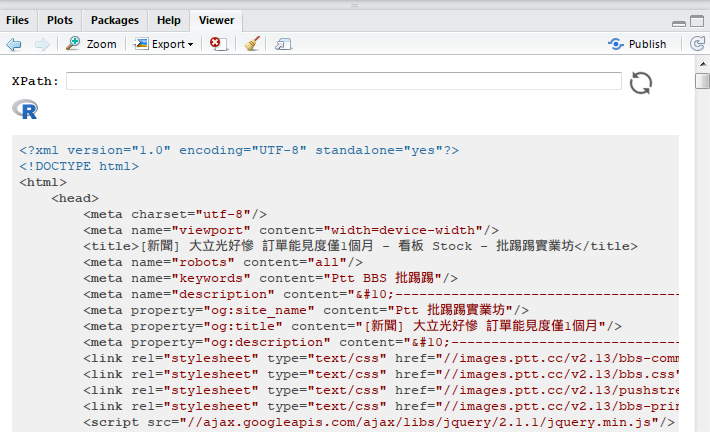``````xml_find_all(doc, '//span[@class="f3 push-content"]', ns=xml2::xml_ns(doc))

## or you want to use rvest package
# doc %>% rvest::html_nodes(xpath = '//span[@class="f3 push-content"]')
``````

``````doc %>%
rvest::html_nodes(xpath = '//span[@class="f3 push-content"]') %>%
rvest::html_text() %>%
`Encoding<-`("UTF-8") %>%
gsub("^: ", "",.)

##  "他，還有隱形眼鏡"
##  "她,還有雙鏡頭"
##  "蘋果怎麼可能容忍供應商EPS180但又不降價？"
##  "它，還有眼鏡蛇"
##  "不要看新聞做股票吧"
##  "外資要壓低吃貨囉"
##  "再爛股價還是會維持四位數啦"
``````

## [R crawler] 公開資訊觀測站 (實作篇)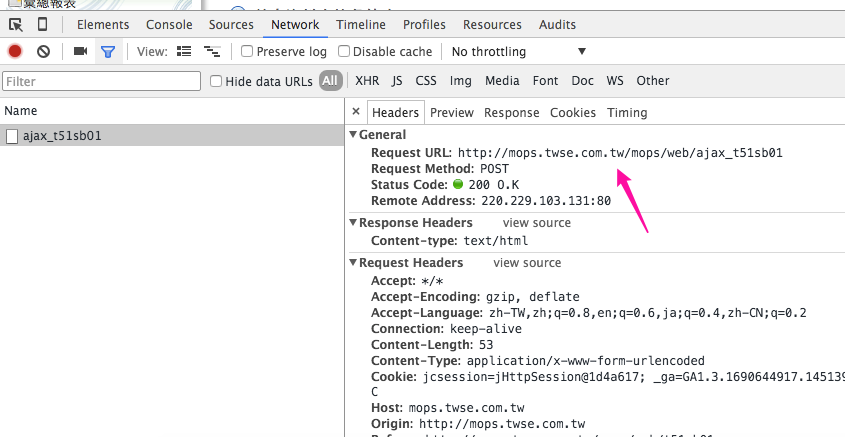``````library(magrittr)
library(httr)
library(rvest)
library(dplyr) # data manipulation & pipe line
library(stringr)
``````

## Connection

``````res <- POST(
"http://mops.twse.com.tw/mops/web/ajax_t51sb01",
body = "encodeURIComponent=1&step=1&firstin=1&TYPEK=sii&code=01",
encode = "form")
``````

``````encodeURIComponent: 1
step: 1
firstin: 1
TYPEK: sii
code:
``````

``````res <- POST(
"http://mops.twse.com.tw/mops/web/ajax_t51sb01",
body = list(
encodeURIComponent = 1,
step = 1,
firstin = 1,
TYPEK = "sii",
code = "01"
),
encode = "form"
)
# ?httr::POST
``````

``````print(res)
``````
``````# Response [http://mops.twse.com.tw/mops/web/ajax_t51sb01]
#   Date: 2016-01-06 13:34
#   Status: 200
#   Content-Type: text/html
#   Size: 18.2 kB
``````

## Parsing

``````res_text <- content(res, "text", encoding = "UTF-8") %>%
`Encoding<-`("UTF-8")  # Windows encodind issue
res_text
``````
`````` "\r\n\r\n<html>\r\n<head>\r\n\t<title>公開資訊觀測站</title>\r\n<!--\t<link href=\"css/css1.css\" rel=\"stylesheet\" type=\"text/css\" Media=\"Screen\"/> -->\r\n<!--\t<script type=\"text/javascript\" src=\"js/mops1.js\"></script> -->\r\n</head>\r\n\r\n<body>\r\n<table class='noBorder'>\n<tr><td align='right'>\n<form action='/server-java/t56ques' method='post'>\n<input type='hidden' name='step' value='0'>\n<input type='hidden' name='Market' value='sii'>\n<input type='hidden' name='SysName' value='公司彙總報表'>\n<input type='hidden' name='reportName' value='公司基本資料查詢彙總表'>\n<input type='hidden' name='colorchg' value=''>\n<input type='hidden' name='QNum' value='1'>\n<input type='hidden' name='Q1N' value='公司類別'>\n<input type='hidden' name='Q1V' value='???d?u·~                '>\n</form></td></tr></table>...
``````

### Parsing html table

`rvest` 套件中的 `html_table()` 可輕鬆幫我們擷取表格。首先先用 `read_html()` 將 html 字串轉成 `xml_document`，再用 `html_nodes()` 選到我們要的表格，最後再將表格擷取出來：

``````res_text <- content(res, as = "text", encoding = "UTF-8")
dt <- res_text %>%
html_nodes(xpath = "//table") %>%
.[]
``````

``````## Windows
dt <- res_text %>%
html_nodes(xpath = "//table") %>%
as.character %>%
.[]
``````

``````View(dt)
``````

## Refactor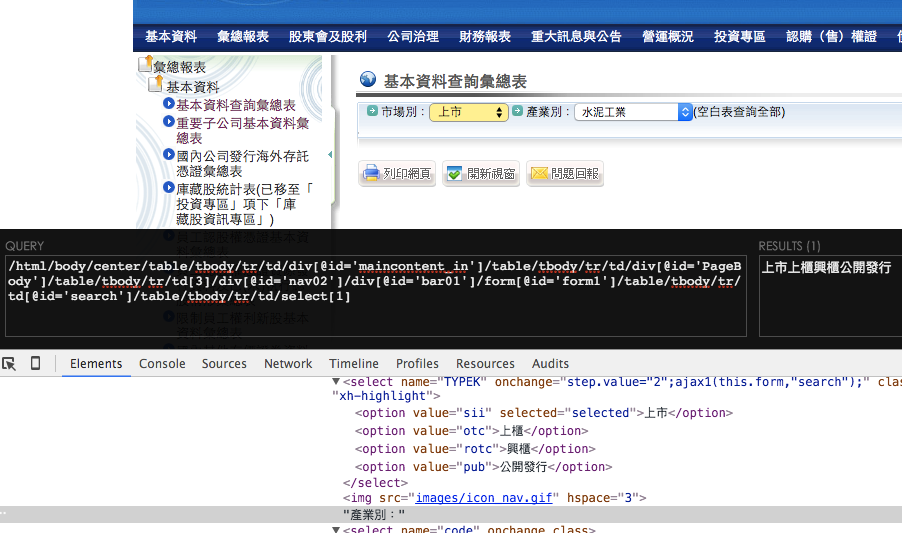``````# different post data values

res_doc <- GET("http://mops.twse.com.tw/mops/web/t51sb01") %>%
content(type="text", encoding = "UTF-8") %>%

market_type <- setNames(
res_doc %>%
html_node(xpath = "//select[@name='TYPEK']") %>%
html_children %>%
html_attr("value"),
res_doc %>%
html_node(xpath = "//select[@name='TYPEK']") %>%
html_children %>%
html_text()
)

market_type
#    上市     上櫃     興櫃 公開發行
#   "sii"    "otc"   "rotc"    "pub"
``````
``````industry_type <- setNames(
res_doc %>%
html_node(xpath = "//select[@name='code']") %>%
html_children %>%
html_attr("value"),
res_doc %>%
html_node(xpath = "//select[@name='code']") %>%
html_children %>%
html_text()
)[-1]

industry_type
# 水泥工業         食品工業         塑膠工業         紡織纖維
# "01"             "02"             "03"             "04"
# 電機機械         電器電纜         化學工業       生技醫療業
# "05"             "06"             "21"             "22"
# 化學生技醫療         玻璃陶瓷         造紙工業         鋼鐵工業
# "07"             "08"             "09"             "10"
# 橡膠工業         汽車工業         半導體業 電腦及週邊設備業
# "11"             "12"             "24"             "25"
# 光電業       通信網路業     電子零組件業       電子通路業
# "26"             "27"             "28"             "29"
# 資訊服務業       其他電子業         電子工業       油電燃氣業
# "30"             "31"             "13"             "23"
# 建材營造           航運業         觀光事業       金融保險業
# "14"             "15"             "16"             "17"
# 貿易百貨         綜合企業             其他         存託憑證
# "18"             "19"             "20"             "91"

``````

``````# get different data
res <- POST(
"http://mops.twse.com.tw/mops/web/ajax_t51sb01",
body = list(
encodeURIComponent = "1",
step = "1",
firstin = "1",
TYPEK = market_type["上櫃"],
code = industry_type["半導體業"]
),
encode = "form"
)

res_text <- content(res, "text", encoding = "UTF-8")
dt2 <- res_text %>%
html_nodes(xpath = "//table") %>%
.[]
``````

## Data Cleansing

``````## data cleansing
dt2 <- dt2 %>%
filter(`公司代號` != "公司代號") %>%
sapply(str_trim)
``````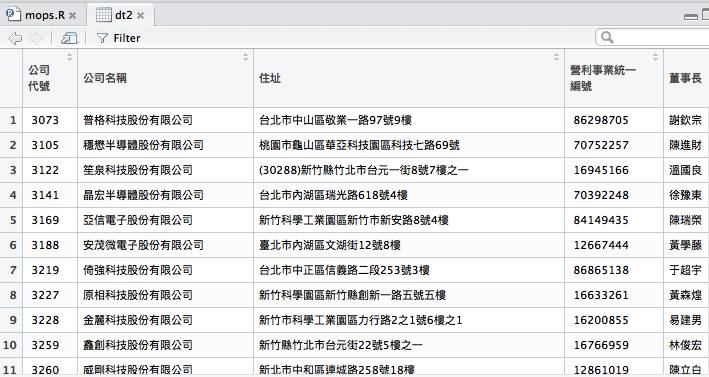## [R crawler] 公開資訊觀測站 (觀察篇)

「⋯⋯以前許多資料源取得的限制， 不再是個無解的難題之後，會釋放出更自由的想像空間，更大的挑戰便是整合資訊的應用，以及如何從中淘金了。」

### Step 1. 定義目標資料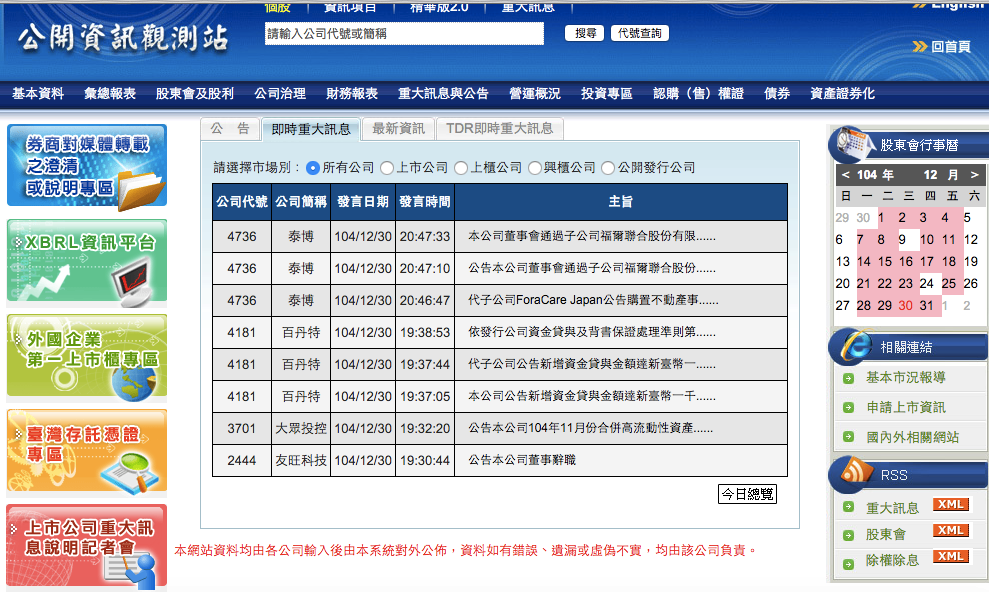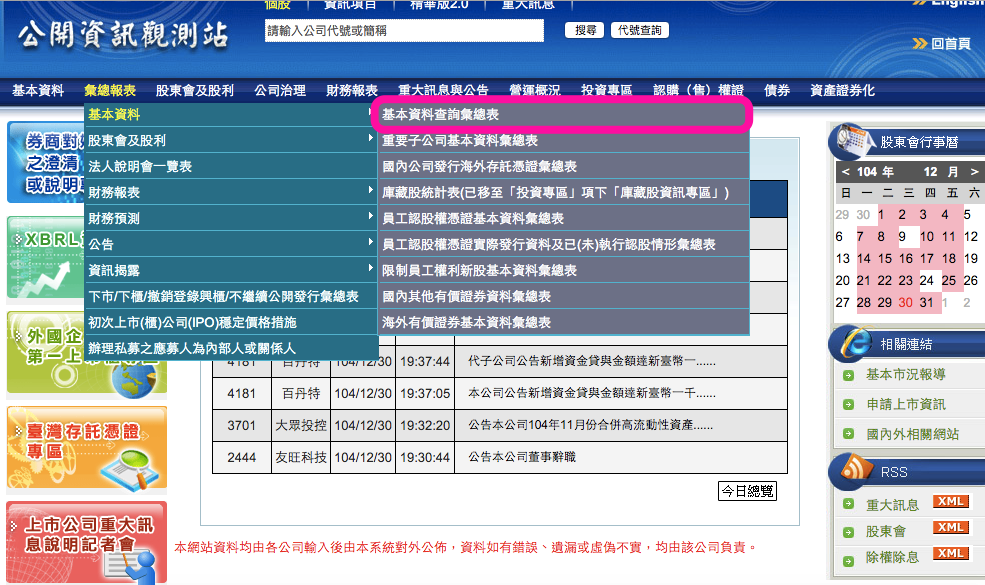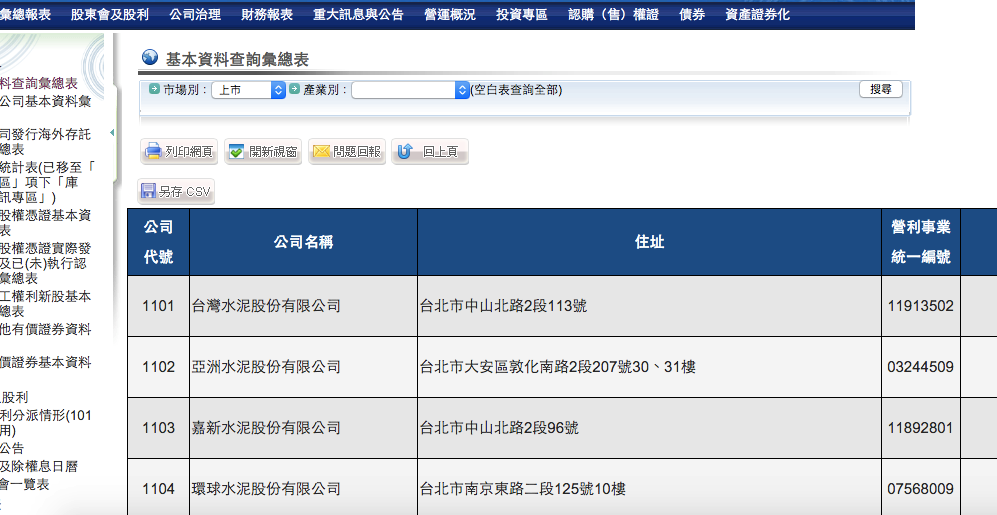### Step 2. 觀察

#### » 找到資料

Tips 可以先按紅色按鈕旁的鈕 清掉之前的連線，在按下「搜尋」按鈕跑出資料的同時，我們需要的那個連線便會出現在前幾個。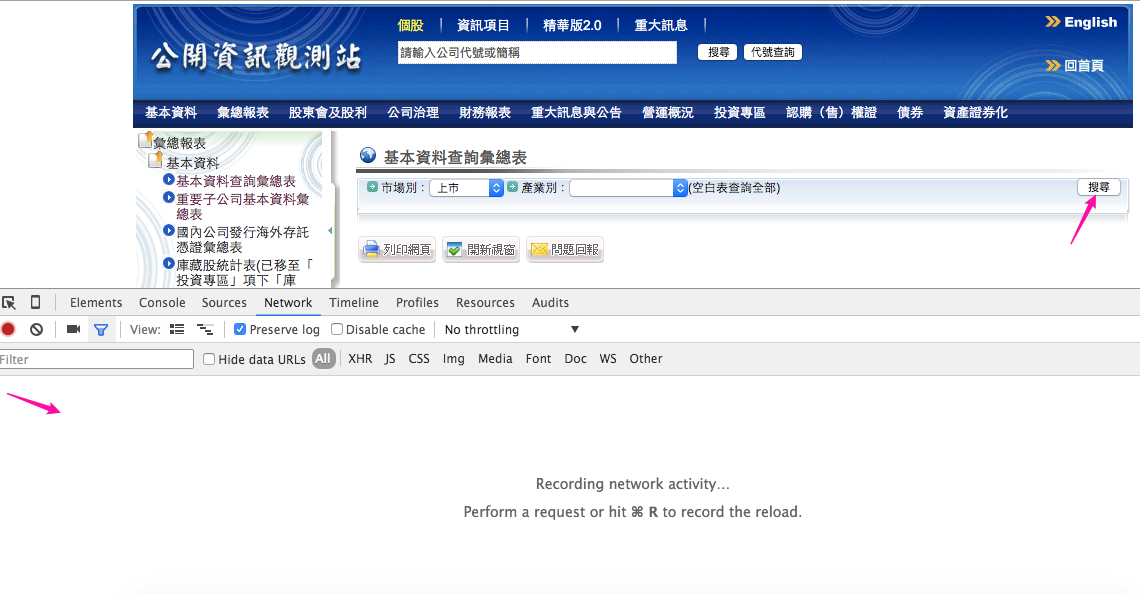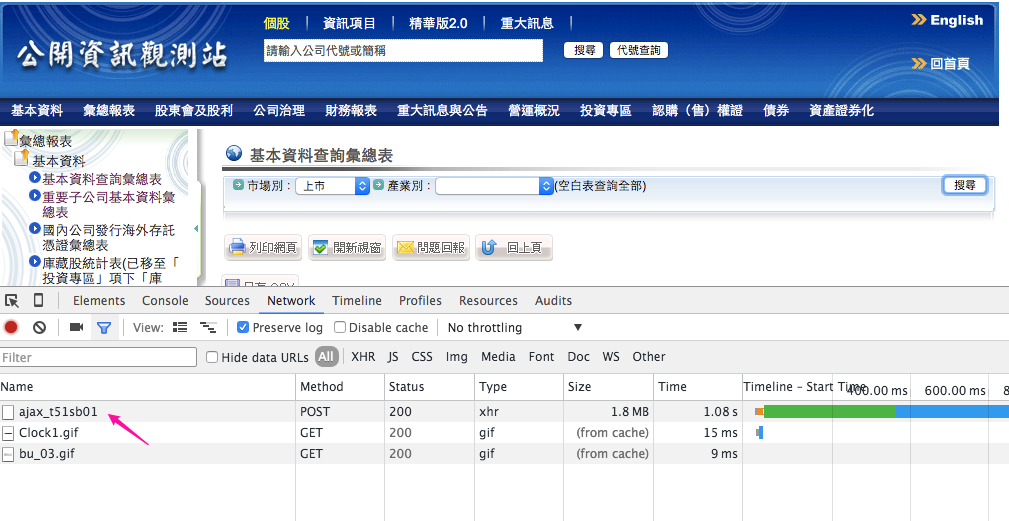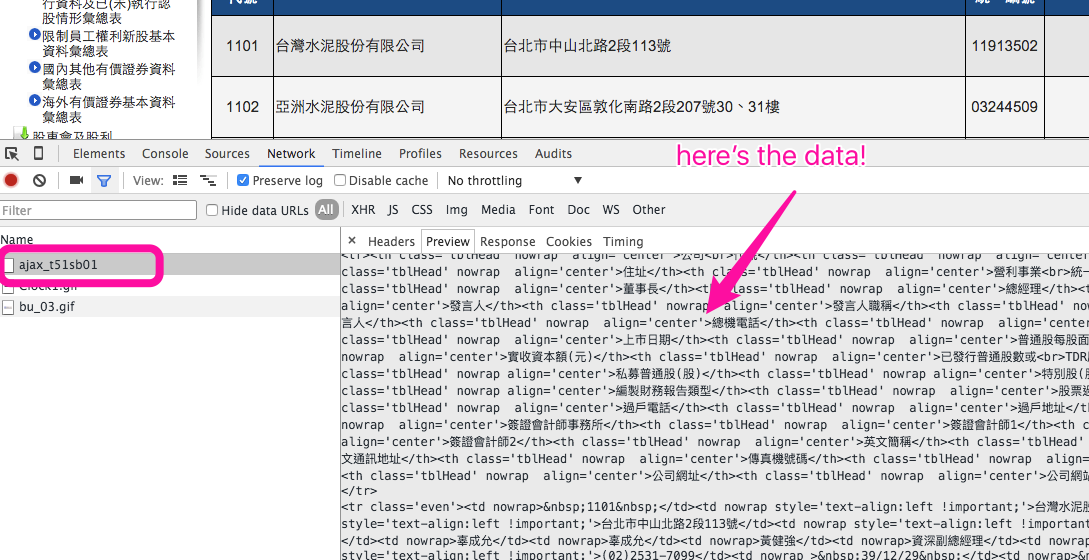#### » 連線方法

http://mops.twse.com.tw/mops/web/ajax_t51sb01``````encodeURIComponent: 1
step: 1
firstin: 1
TYPEK: sii
code:
``````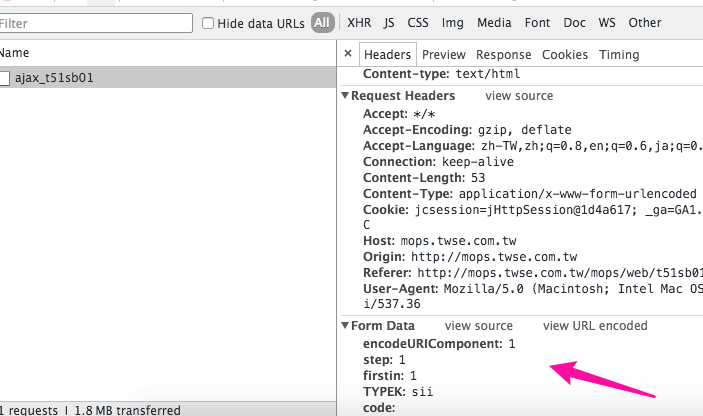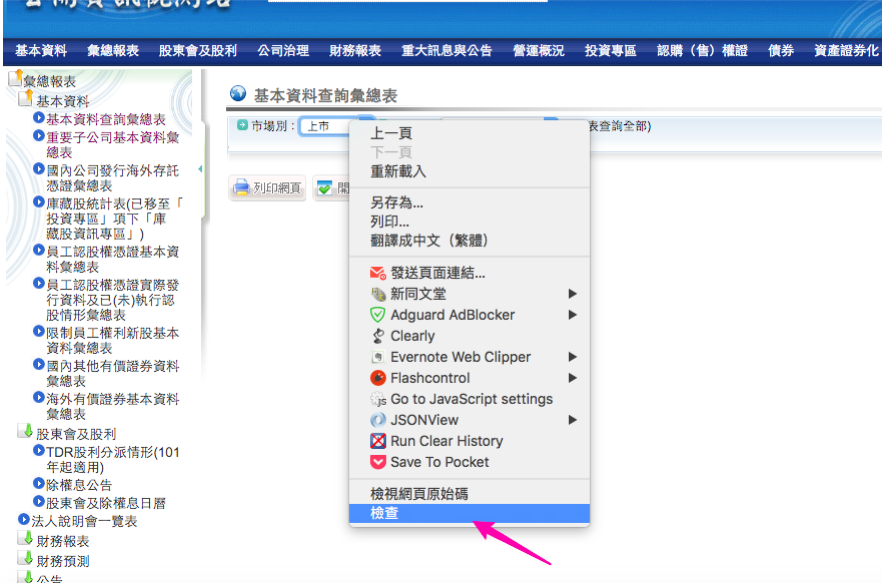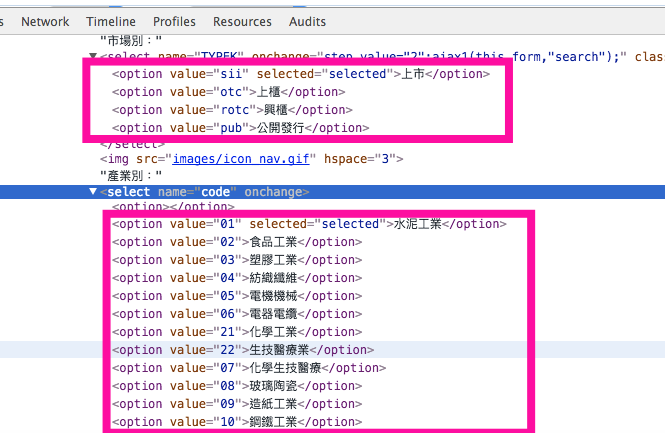``````TYPEK: sii
code:
``````